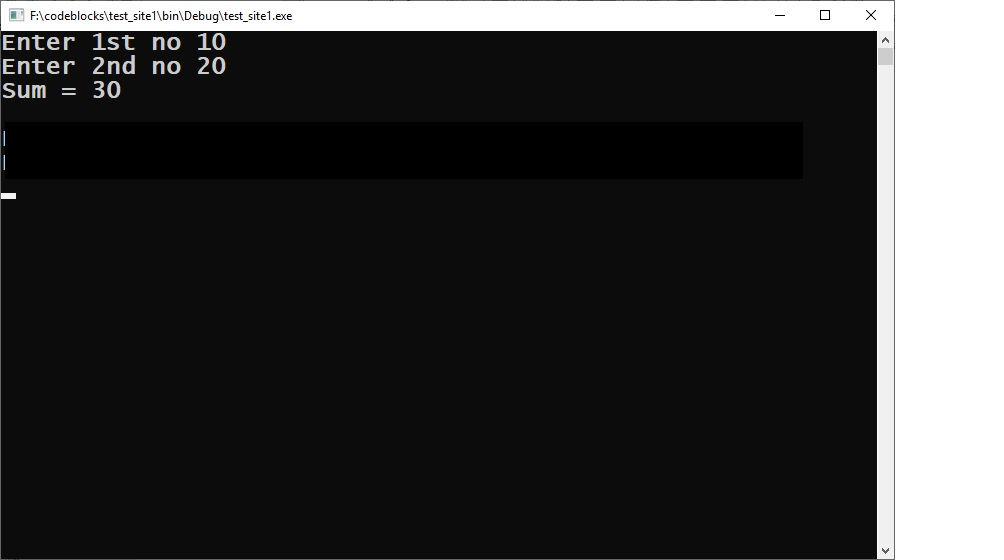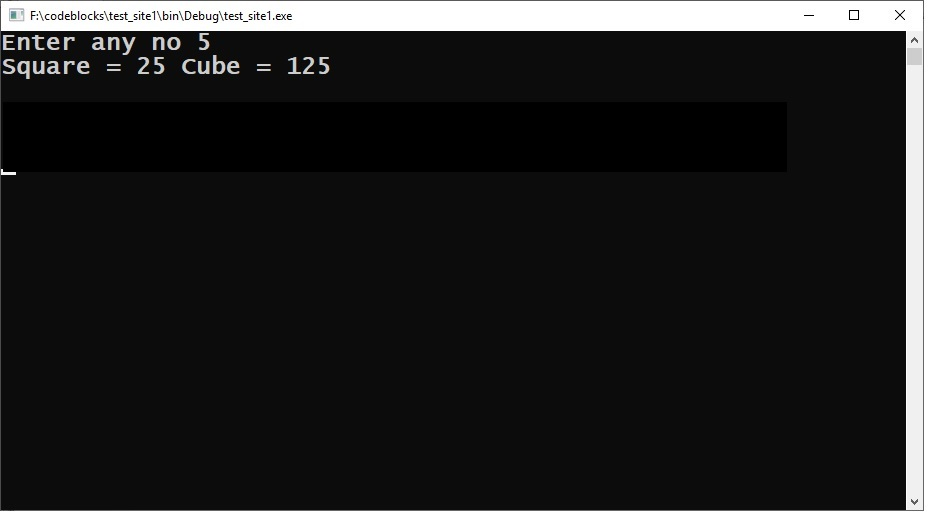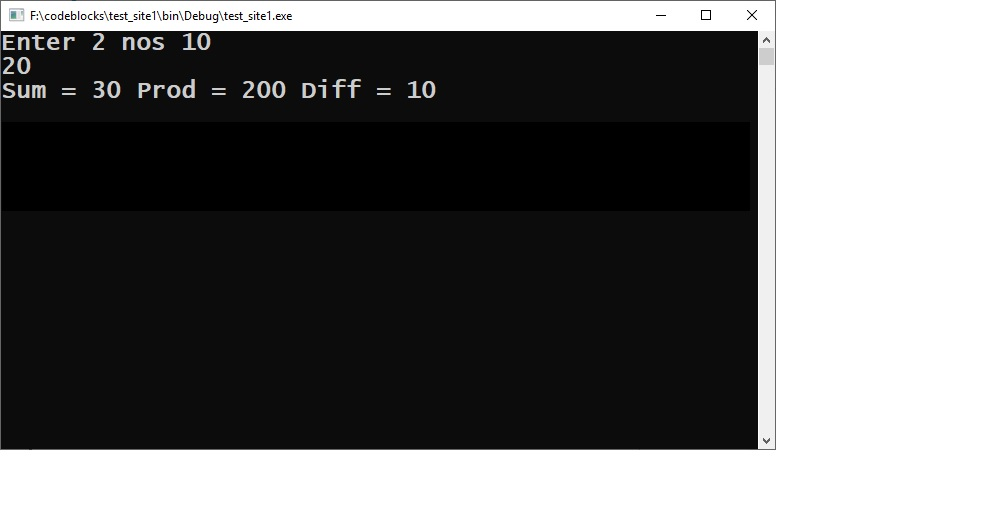#### C++ Basic Programs

C++ Basic Programs Set 1

C++ Basic Programs Set 2

#### File Handling

Examples Set 1(Q 1 to4)

Examples Set 2(Q 5 to 7)

Example Set 3(Q8 to 10)

Example Set 4(Q11 to 12)

Example Set 5(Q13 to 15)

Examples Set 6(Q16 to )

# Cpp basic programs Set 1

c++ basic programs set 1

Q. 1.) Write a C++ Program to take input for two numbers , calculate and print their sum?#include <iostream>

using namespace std;

int main()
{
int a,b,c;
cout<<“Enter 1st no “;
cin>>a;
cout<<“Enter 2nd no “;
cin>>b;
c=a+b;
cout<<“Sum = “<<c<<endl;
return 0;
}

Q.2.) Write a C++ Program to take input for a number calculate and print its square and cube?#include <iostream>

using namespace std;

int main()
{
int a,b,c;
cout<<“Enter any no “;
cin>>a;
b=a*a;
c=a*a*a;
cout<<“Square = “<<b<<” Cube = “<<c<<endl;
return 0;
}

Q.3.) Write a C++ Program to take input for two numbers calculate and print their sum, product and diff ?#include <iostream>

using namespace std;

int main()
{
int a,b,s,p,d;
cout<<“Enter 2 nos “;
cin>>a>>b;
s=a+b;
p=a*b;
if(a>b)
d=a-b;
else
d=b-a;
cout<<“Sum = “<<s<<” Prod = “<<p<<” Diff = “<<d<< endl;
return 0;
}

Important PagesNumber Of Visitors